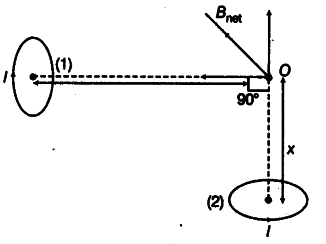# Two small identical circular loops marked 1 and 2 carrying equal currents are placed with the geometrical axis perpendicular to each other as shown in figure. Find the magnitude and direction of the net magnetic field produced at the point O.Dear Student
The magnetic field at a point due to a circular loop is given by

where, / = current through the loop
a = radius of the loop
r = distance of O from the center of the loop.
Since I, a and r = x are the same for both the loops, the magnitude of B will be the same and is given by

The direction of magnetic field due to loop (1) will be away from 0 and that of the magnetic field due to loop (2) will be towards O as shown. The direction of the net magnetic field will be as shown below:The magnitude of the net magnetic field is given by

Regards

• 0
What are you looking for?